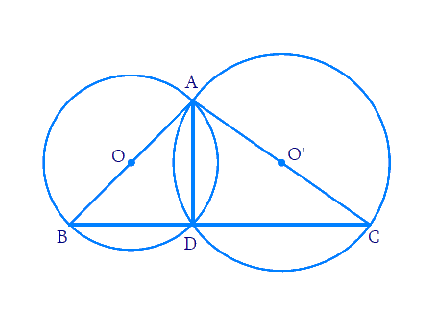# Ex.10.5 Q10 Circles Solution - NCERT Maths Class 9

Go back to  'Ex.10.5'

## Question

If circles are drawn taking two sides of a triangle as diameters, prove that the point of intersection of these circles lie on the third side.

Video Solution
Circles
Ex 10.5 | Question 10

## Text Solution

What is given ?

Two circles are drawn taking two sides of a triangle as diameters.

What is unknown?

To prove  that point of intersection of the $$2$$ circles lie on the third side.

Reasoning:

Angle in a semicircle is a right angle. By using this fact we can show that $$BDC$$ is a line which will lead to the proof that point of intersection lie on the third side.

Steps:Since angle in a semicircle is a right angle, we get:

\begin{align} \angle {ADB}&=90^{\circ} \text { and } \angle {ADC}=90^{\circ} \end{align}

\begin{align} \angle {ADB}+\angle {ADC}&=90^{\circ}+90^{\circ} \\ \Rightarrow \;\; \angle {ADB}+\angle {ADC}&=180^{\circ} \end{align}

$$\Rightarrow \quad{BDC} \text { is a straight line. }$$

∴ $${D}$$ lies on $${BC}$$

Hence, point of intersection of circles lie on the third side $$BC.$$

Video Solution
Circles
Ex 10.5 | Question 10

Learn from the best math teachers and top your exams

• Live one on one classroom and doubt clearing
• Practice worksheets in and after class for conceptual clarity
• Personalized curriculum to keep up with school